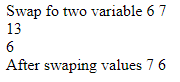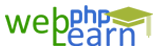Apply for Zend Framework Certification Training

# Php Questions\$a = 6;
\$b = 7;
\$temp = \$a;
\$a = \$b;
\$b = \$temp;
echo "Number a =".\$a." and b=".\$b."\n"
\$a = 6;
\$b = 7;
list(\$a, \$b) = array(\$b, \$a);
print \$a . \',\' . \$b;
\$a = \$a + \$b;
\$b = \$a - \$b;
\$a = \$a - \$b;
print \$a . \',\' . \$b;

`{{questionlistdata.blog_question_description}}`
`{{answer.blog_answer_description  }}`